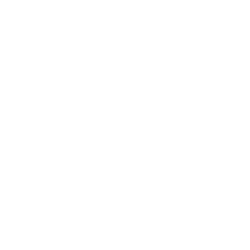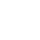## Manipulate an Image with Scripting`docRef_1.activeLayer.applyGaussianBlur(borderSize / 3); docRef_1.activeLayer.opacity = 50;`

The first line is in the same for as the average blur which we made in step 5.

### Step 17:

We now want the script to rotate both the image and the shadow then merge them, this gives a better result than merging them then rotating. We want the angle of rotation to be different each time but somewhere between -20° and 20°. The code for this is:

`var angle = Math.round(Math.random() * 40) - 20; docRef_1.activeLayer.rotate(angle, AnchorPosition.MIDDLECENTER); docRef_1.activeLayer = docRef_1.layers; docRef_1.activeLayer.rotate(angle, AnchorPosition.MIDDLECENTER); docRef_1.activeLayer.merge();`

The Math.random function will select a number between 0 and 1, so this number is not an integer, this is why we have the rounding function in the first line. To manipulate this number so it is between -20 and 20 we have the script multiply it by 40 then subtract 20 from the rounded number. This number is stored in a variable named angle.

We then have the rotate command used on the active layer which is still the shadow layer, her define the angle to be our angle variable and the anchor to be in the middle. The rotation can be done in Photoshop by hitting Ctrl+T.

The last three lines select the image layer, rotate it then merge them.

### Final Script:

```preferences.rulerUnits = Units.PIXELS; displayDialogs = DialogModes.NO

open(File(openDialog())); var docRef_1 = activeDocument; docRef_1.backgroundLayer.duplicate(); var white = new SolidColor(); white.rgb["hexValue"] = "ffffff" var black = new SolidColor(); black.rgb["hexValue"] = "000000" foregroundColor = black; backgroundColor = white; docRef_1.selection.selectAll(); docRef_1.selection.fill(white); docRef_1.selection.deselect(); docRef_1.layers.duplicate(); docRef_1.activeLayer = docRef_1.layers; docRef_1.activeLayer.applyAverage(); for (RLevel = 0; RLevel <= 255; RLevel ++) { if (docRef_1.channels["Red"].histogram[RLevel]) { break; } } for (GLevel = 0; GLevel <= 255; GLevel ++) { if (docRef_1.channels["Green"].histogram[GLevel]) { break; } } for (BLevel = 0; BLevel <= 255; BLevel ++) { if (docRef_1.channels["Blue"].histogram[BLevel]) { break; } } var R = 0; var G = 0; var B = 0; if (RLevel > GLevel && RLevel > BLevel) { R = 255; } if (GLevel > BLevel && GLevel > RLevel) { G = 255; } if (BLevel > RLevel && BLevel > GLevel) { B = 255; } var color = new SolidColor(); color.rgb.red = R; color.rgb.green = G; color.rgb.blue = B; docRef_1.selection.selectAll(); docRef_1.selection.fill(color); docRef_1.selection.deselect(); docRef_1.activeLayer.invert(); docRef_1.activeLayer.blendMode = BlendMode.COLORBLEND; docRef_1.activeLayer.opacity = 50; docRef_1.activeLayer.merge(); var width = docRef_1.width; var height = docRef_1.height; if (width <= height) { var borderSize = Math.round(width * 0.15); } else { var borderSize = Math.round(height * 0.15); } docRef_1.resizeCanvas(width + borderSize, height + borderSize); docRef_1.artLayers.add(); docRef_1.activeLayer.move(docRef_1.backgroundLayer, ElementPlacement.PLACEBEFORE); docRef_1.selection.selectAll(); docRef_1.selection.fill(white); docRef_1.selection.deselect(); docRef_1.layers.merge(); docRef_1.artLayers.add(); docRef_1.activeLayer.move(docRef_1.backgroundLayer, ElementPlacement.PLACEBEFORE); docRef_1.selection.selectAll(); docRef_1.selection.fill(black); docRef_1.selection.deselect(); var width = docRef_1.width; var height = docRef_1.height; var newSize = Math.round(Math.sqrt(width * width + height * height)) + borderSize; docRef_1.resizeCanvas(newSize, newSize); docRef_1.activeLayer.applyGaussianBlur(borderSize / 3); docRef_1.activeLayer.opacity = 50; var angle = Math.round(Math.random() * 40) - 20; docRef_1.activeLayer.rotate(angle, AnchorPosition.MIDDLECENTER); docRef_1.activeLayer = docRef_1.layers; docRef_1.activeLayer.rotate(angle, AnchorPosition.MIDDLECENTER); docRef_1.activeLayer.merge();```

Pages: 1 2 3 4 5 6 7 8 9

### One comment on “Manipulate an Image with Scripting”

1.Drake says:

confused